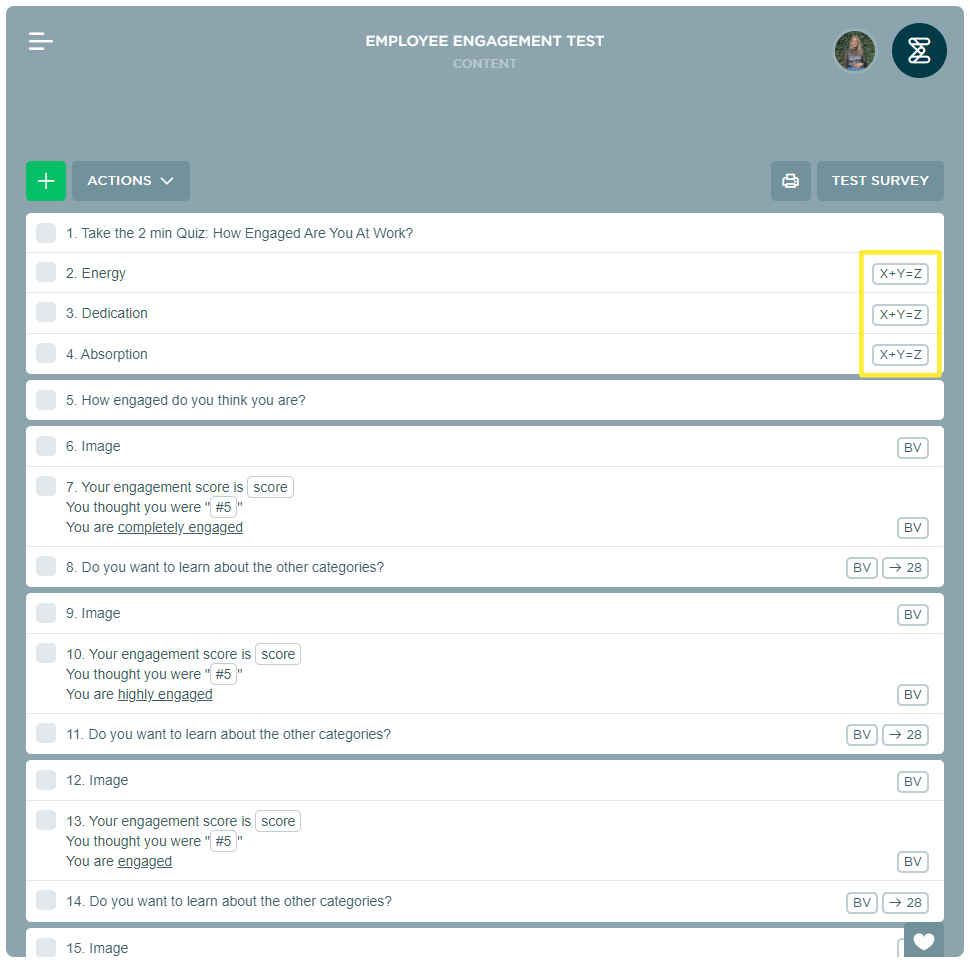# Score Calculations

Score Calculations is the perfect feature for making quizzes, online order forms, and more. This feature gives you the ability to apply a calculation to the response options to specific question types, i.e. additions, subtractions, multiplications, and divisions can be made throughout the entire survey. These calculations will give your respondent a final score at the end of the survey. This means you can total up quiz or test results, as well as prices for order forms.

## Questions Compatible with Score Calculations

The following question types are compatible with the Score Calculations feature:

To add Score Calculations to your survey, all you have to do is:

1. Go to your survey and click the relevant question.
2. Click on settings and enable the calculations setting.
3. The following steps differ depending on the question type.
1. The following applies to Multiple Choice, Rating Scale, Image Choice, Net Promoter Score®, and Recommendation Score questions.

1. Click on setup calculations.
2. Click on the enable calculations dropdown menu and select the response option you want to add the calculation to.
3. From the calculations dropdown menu, you can choose between the following operations: add, subtract, multiply and divide.
4. Once you've selected the operation, add a value. For example, if you selected "add" as the operation, the value you insert will be added to the respondent's score if they select that response option.
5. You can add as many calculations as you need by repeating these steps.
6. When you're done click save.
2. The following applies to Matrix Multiple Choice and Matrix Rating Scale questions.

1. Click on the back arrow () to go back to the sub-questions.
2. Click on the sub-question that you want to add calculations to.
3. Click on setup calculations.
4. From the calculations dropdown menu, you can choose between the following operations: add, subtract, multiply and divide.
5. Once you've selected the operation, add a value. For example, if you selected "add" as the operation, the value you insert will be added to the respondent's score if they select that response option.
6. You can add as many calculations as you need by repeating these steps.
7. When you're done click save.
4. You are always able to see which questions contain calculations from the survey content overview:## Display the Score for Respondents

You can show respondents the score throughout as well as at the end of the survey by using merge fields.

All you have to do is:

1. Go to your survey and click on the text element, question, or end page where you want to display the score.
2. Click on the question or text box and click the merge field dropdown menu.3. Select the score variable from the dropdown.
4. This will merge the respondent score in the corresponding text element or question.